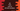# C# program to find the sum of all even numbers below a given number## C# program to find the sum of all even numbers below a given number:

In this C# program, we will learn how to find the sum of all even numbers in a range from 1 to n. We will take the value of n as input from the user and print the sum of all even numbers.

The easiest way to find the sum of all even numbers below a given number is by using a loop. We can write one loop that will run from 1 to n, check for each number if it is even or not and if it is, add it to a sum variable. The sum variable is initialized as 0 and it will hold the total sum once the loop ends.

### Method 1: Find the sum of all even numbers below a given number using a for loop:

Let’s try to use a for loop to find the sum of all even numbers below a given number:

``````using System;

public class Program {

public static void Main(string[] args) {
int i, num, sum = 0;
Console.WriteLine("Enter a number :");

for(i = 2; i<= num; i++){
if(i % 2 == 0){
sum += i;
}
}

Console.WriteLine("Total sum of all even numbers less than "+num+" is: "+sum);
}
}``````

Here,

• We have initialized three integer variables i, num and sum. i is to use with the for loop, num to store the user input number and sum to hold the sum of the even numbers.
• It is reading the number entered by the user and storing it in the variable num.
• The for loop runs from 2 to num and for each value it finds, it checks for if it is even or not. If it is, it is adding that value to sum.

It will print output as like below:

``````Enter a number :
20
Total sum of all even numbers less than 20 is: 110``````

### Method 2: Improving the for loop without checking for even:

We can also increment the value of i by 2 in the for loop. That will make it easier and we don’t have to check if the current number is divisible by 2 or not.

``````using System;

public class Program {

public static void Main(string[] args) {
int i, num, sum = 0;
Console.WriteLine("Enter a number :");

for(i = 2; i< num; i +=2){
sum += i;
}

Console.WriteLine("Total sum of all even numbers less than "+num+" is: "+sum);
}
}``````

It will print a similar output:

``````Enter a number :
20
Total sum of all even numbers less than 20 is: 110``````

### Method 3: Using a while loop:

We can also use a while loop to do the same thing.

``````using System;

public class Program {

public static void Main(string[] args) {
int i = 2, num, sum = 0;
Console.WriteLine("Enter a number :");

while(i <= num){
sum += i;
i += 2;
}

Console.WriteLine("Total sum of all even numbers less than "+num+" is: "+sum);
}
}``````

In this program,

• We are using a while loop that runs from i = 2 to i = num.
• Inside the while loop, it is incrementing the value of i by 2 on each iteration.
• It is incrementing the value of sum on each iteration.

It will give similar output.

``````Enter a number :
20
Total sum of all even numbers less than 20 is: 110``````